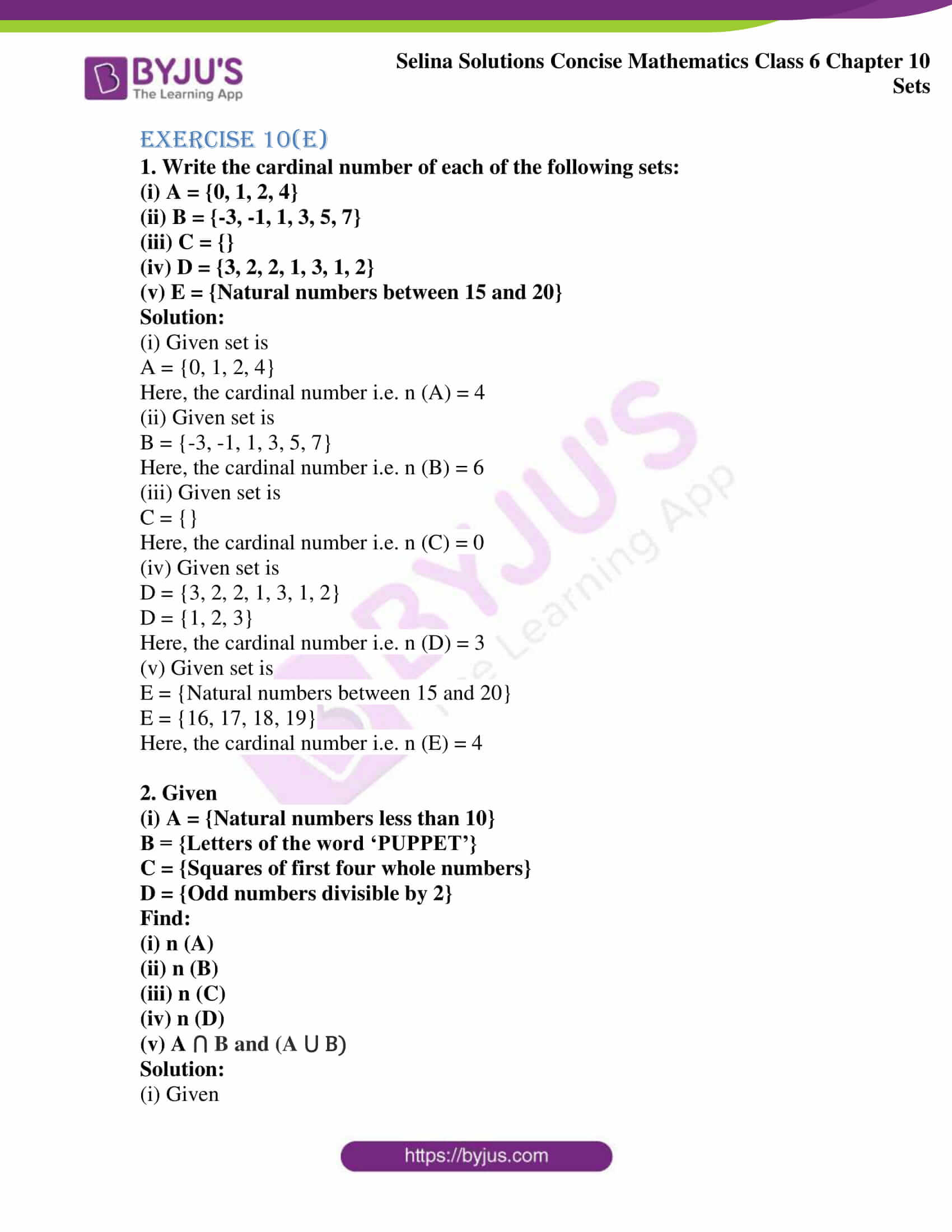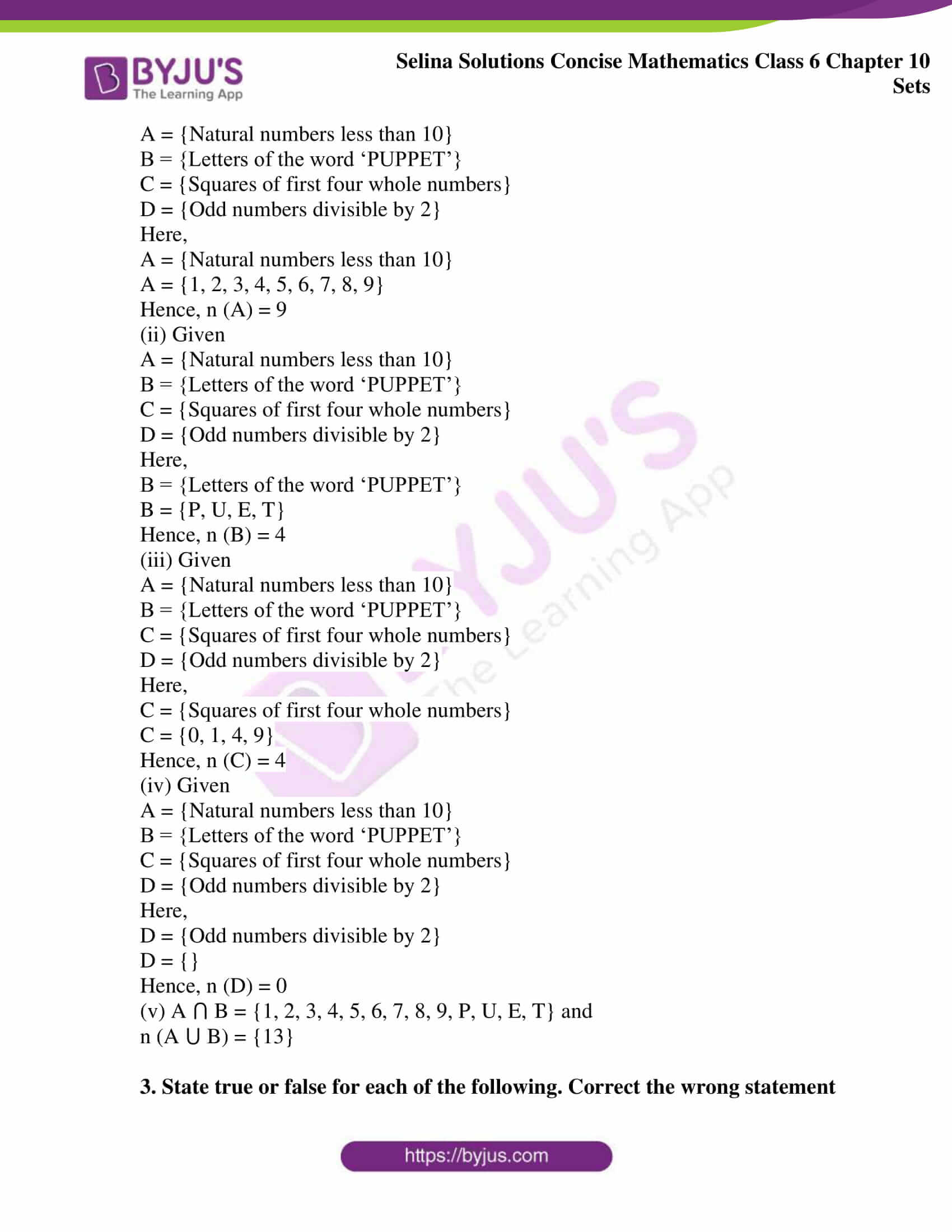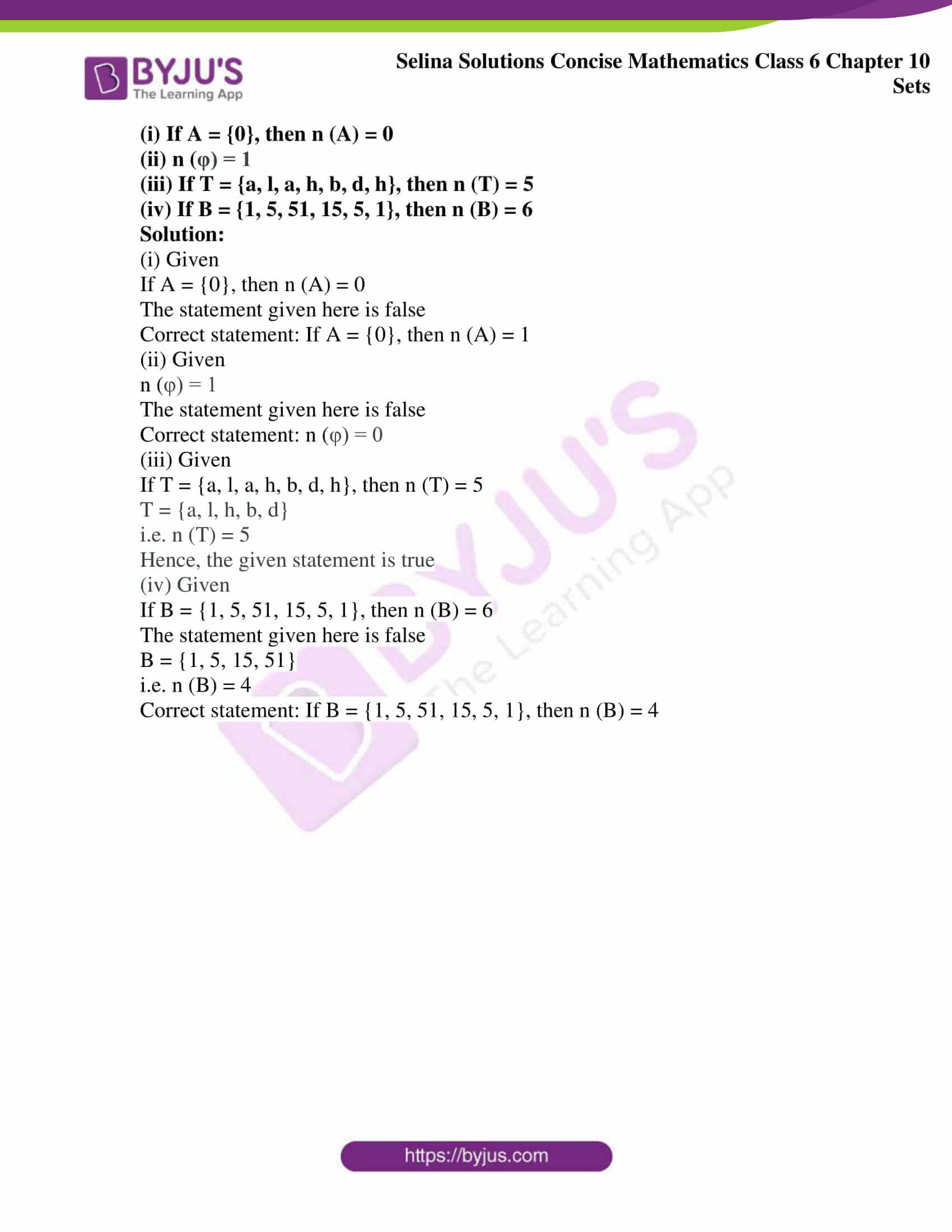# Selina Solutions Concise Mathematics Class 6 Chapter 10: Sets Exercise 10(E)

Selina Solutions Concise Mathematics Class 6 Chapter 10: Sets Exercise 10(E) discusses concepts related to cardinal number. Students can refer to Selina Solutions to secure good marks in the board examination. Expert tutors have designed the solutions with the intention to help students understand the concepts well. Students who aspire to come out with flying colours in the final exams are advised to practice these solutions meticulously. Selina Solutions Concise Mathematics Class 6 Chapter 10 Sets Exercise 10(E) PDF are available from the links given below, which can be downloaded free and can be used as a reference guide in future.

## Selina Solutions Concise Mathematics Class 6 Chapter 10: Sets Exercise 10(E) Download PDF### Access other exercises of Selina Solutions Concise Mathematics Class 6 Chapter 10: Sets

Exercise 10(A) Solutions

Exercise 10(B) Solutions

Exercise 10(C) Solutions

Exercise 10(D) Solutions

### Access Selina Solutions Concise Mathematics Class 6 Chapter 10: Sets Exercise 10(E)

#### Exercise 10(E)

1. Write the cardinal number of each of the following sets:

(i) A = {0, 1, 2, 4}

(ii) B = {-3, -1, 1, 3, 5, 7}

(iii) C = {}

(iv) D = {3, 2, 2, 1, 3, 1, 2}

(v) E = {Natural numbers between 15 and 20}

Solution:

(i) Given set is

A = {0, 1, 2, 4}

Here, the cardinal number i.e. n (A) = 4

(ii) Given set is

B = {-3, -1, 1, 3, 5, 7}

Here, the cardinal number i.e. n (B) = 6

(iii) Given set is

C = {}

Here, the cardinal number i.e. n (C) = 0

(iv) Given set is

D = {3, 2, 2, 1, 3, 1, 2}

D = {1, 2, 3}

Here, the cardinal number i.e. n (D) = 3

(v) Given set is

E = {Natural numbers between 15 and 20}

E = {16, 17, 18, 19}

Here, the cardinal number i.e. n (E) = 4

2. Given

(i) A = {Natural numbers less than 10}

B = {Letters of the word ‘PUPPET’}

C = {Squares of first four whole numbers}

D = {Odd numbers divisible by 2}

Find:

(i) n (A)

(ii) n (B)

(iii) n (C)

(iv) n (D)

(v) A ⋂ B and (A ⋃ B)

Solution:

(i) Given

A = {Natural numbers less than 10}

B = {Letters of the word ‘PUPPET’}

C = {Squares of first four whole numbers}

D = {Odd numbers divisible by 2}

Here,

A = {Natural numbers less than 10}

A = {1, 2, 3, 4, 5, 6, 7, 8, 9}

Hence, n (A) = 9

(ii) Given

A = {Natural numbers less than 10}

B = {Letters of the word ‘PUPPET’}

C = {Squares of first four whole numbers}

D = {Odd numbers divisible by 2}

Here,

B = {Letters of the word ‘PUPPET’}

B = {P, U, E, T}

Hence, n (B) = 4

(iii) Given

A = {Natural numbers less than 10}

B = {Letters of the word ‘PUPPET’}

C = {Squares of first four whole numbers}

D = {Odd numbers divisible by 2}

Here,

C = {Squares of first four whole numbers}

C = {0, 1, 4, 9}

Hence, n (C) = 4

(iv) Given

A = {Natural numbers less than 10}

B = {Letters of the word ‘PUPPET’}

C = {Squares of first four whole numbers}

D = {Odd numbers divisible by 2}

Here,

D = {Odd numbers divisible by 2}

D = {}

Hence, n (D) = 0

(v) A ⋂ B = {1, 2, 3, 4, 5, 6, 7, 8, 9, P, U, E, T} and

n (A ⋃ B) = {13}

3. State true or false for each of the following. Correct the wrong statement

(i) If A = {0}, then n (A) = 0

(ii) n (φ) = 1

(iii) If T = {a, l, a, h, b, d, h}, then n (T) = 5

(iv) If B = {1, 5, 51, 15, 5, 1}, then n (B) = 6

Solution:

(i) Given

If A = {0}, then n (A) = 0

The statement given here is false

Correct statement: If A = {0}, then n (A) = 1

(ii) Given

n (φ) = 1

The statement given here is false

Correct statement: n (φ) = 0

(iii) Given

If T = {a, l, a, h, b, d, h}, then n (T) = 5

T = {a, l, h, b, d}

i.e. n (T) = 5

Hence, the given statement is true

(iv) Given

If B = {1, 5, 51, 15, 5, 1}, then n (B) = 6

The statement given here is false

B = {1, 5, 15, 51}

i.e. n (B) = 4

Correct statement: If B = {1, 5, 51, 15, 5, 1}, then n (B) = 4